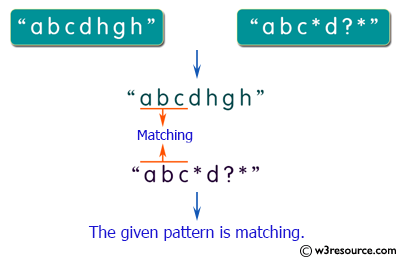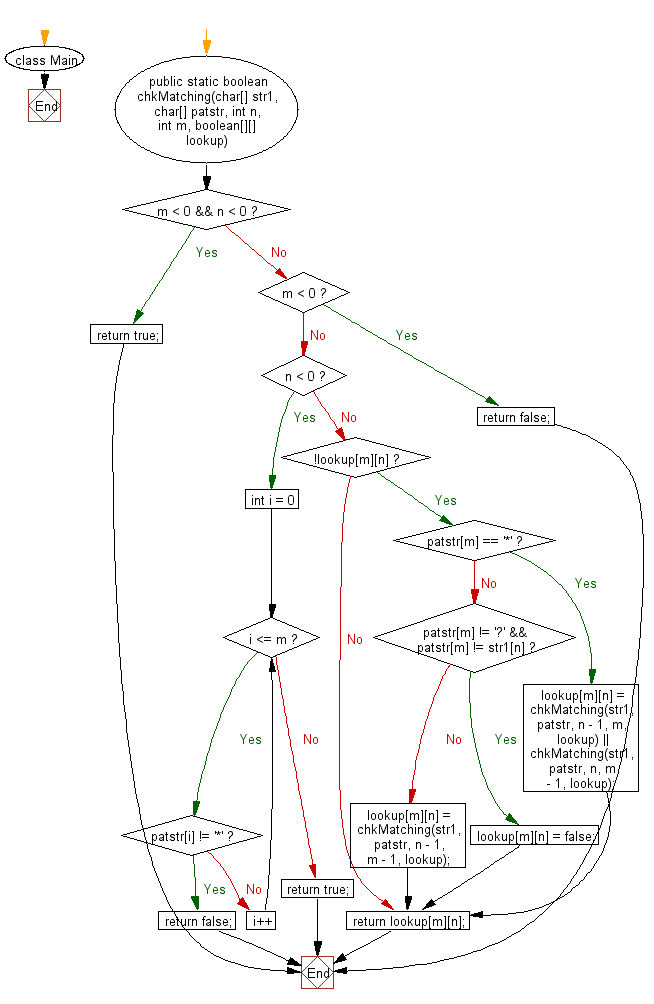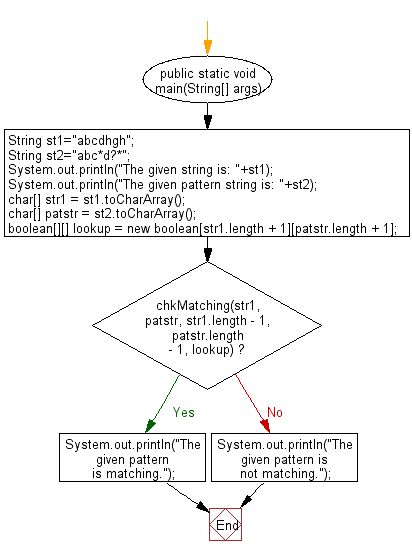﻿ Java exercises: Match two strings where one string contains wildcard characters - w3resource# Java String Exercises: Match two strings where one string contains wildcard characters

## Java String: Exercise-53 with Solution

Write a Java program to match two strings where one string contains wildcard characters.

Sample Solution:

Java Code:

``````import java.util.*;
class Main
{
public static boolean chkMatching(char[] str1, char[] patstr, int n, int m,
boolean[][] lookup)
{
if (m < 0 && n < 0)
{
return true;
}
else if (m < 0)
{
return false;
}
else if (n < 0)
{
for (int i = 0; i <= m; i++)
{
if (patstr[i] != '*')
{
return false;
}
}
return true;
}
if (!lookup[m][n])
{
if (patstr[m] == '*')
{
lookup[m][n] = chkMatching(str1, patstr, n - 1, m, lookup) ||
chkMatching(str1, patstr, n, m - 1, lookup);
}
else
{
if (patstr[m] != '?' && patstr[m] != str1[n])
{
lookup[m][n] = false;
}
else
{
lookup[m][n] = chkMatching(str1, patstr, n - 1, m - 1, lookup);
}
}
}
return lookup[m][n];
}
public static void main(String[] args)
{
String st1="abcdhgh";
String st2="abc*d?*";
System.out.println("The given string is: "+st1);
System.out.println("The given pattern string is: "+st2);
char[] str1 = st1.toCharArray();
char[] patstr = st2.toCharArray();

boolean[][] lookup = new boolean[str1.length + 1][patstr.length + 1];
if (chkMatching(str1, patstr, str1.length - 1, patstr.length - 1, lookup))
{
System.out.println("The given pattern is matching.");
}
else
{
System.out.println("The given pattern is not matching.");
}
}
}
```
```

Sample Output:

```The given string is: abcdhgh
The given pattern string is: abc*d?*
The given pattern is matching.
```

Pictorial Presentation:Flowchart: 1Flowchart: 2Java Code Editor:

Improve this sample solution and post your code through Disqus

What is the difficulty level of this exercise?

﻿